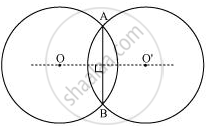# If Two Circles Intersect at Two Points, Prove that Their Centres Lie on the Perpendicular Bisector of the Common Chord. - Mathematics

If two circles intersect at two points, prove that their centres lie on the perpendicular bisector of the common chord.

#### SolutionConsider two circles centered at point O and O’, intersecting each other at point A and B respectively.

Join AB. AB is the chord of the circle centered at O. Therefore, perpendicular bisector of AB will pass through O.

Again, AB is also the chord of the circle centered at O’. Therefore, perpendicular bisector of AB will also pass through O’.

Clearly, the centres of these circles lie on the perpendicular bisector of the common chord.

Concept: Circles Passing Through One, Two, Three Points
Is there an error in this question or solution?

#### APPEARS IN

NCERT Class 9 Maths
Chapter 10 Circles
Exercise 10.3 | Q 3 | Page 176